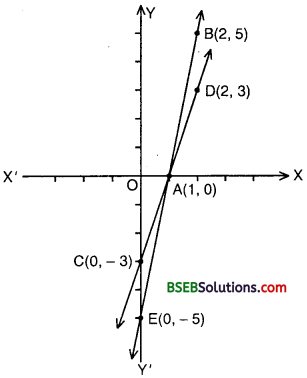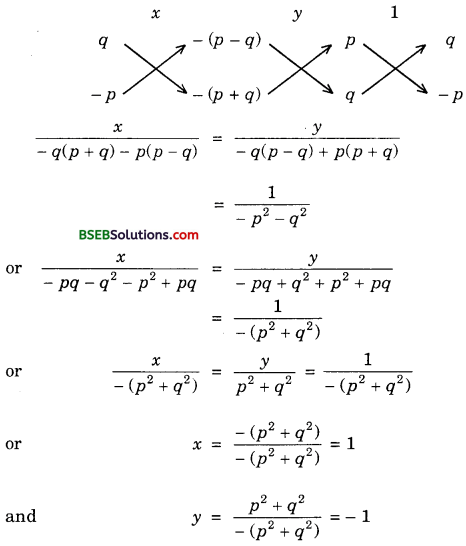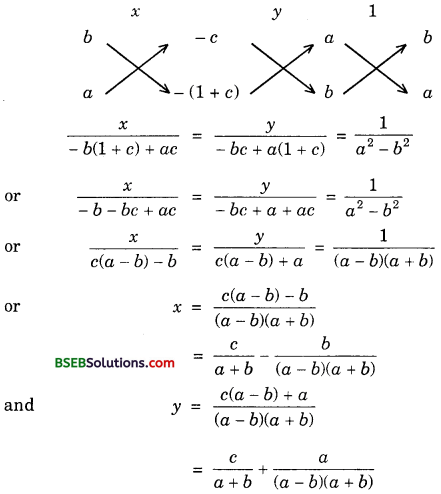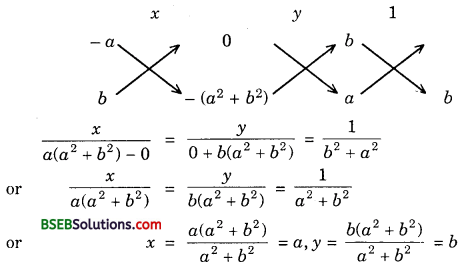# Bihar Board Class 10th Maths Solutions Chapter 3 Pair of Linear Equations in Two Variables Ex 3.7

Bihar Board Class 10th Maths Solutions Chapter 3 Pair of Linear Equations in Two Variables Ex 3.7 Textbook Questions and Answers.

## BSEB Bihar Board Class 10th Maths Solutions Chapter 3 Pair of Linear Equations in Two Variables Ex 3.7

Question 1.
The ages of two friends Ani and Biju differ by 3 years. Ani’s father Dharam is twice as old as Ani and Biju is twice as old as his sister Cathy.
The ages of Cathy and Dharam differ by 30 years. Find the ages of Ani and Biju?
Solution:
Let the ages of Ani and Biju be x years and y years respectively. Then,
x – y = ±3 [Given]
Dharam’s age = 2x, and Cathy’s age = $$\frac{y}{2}$$ Clearly, Dharam is older than Cathy.
Thus, we have the following two systems of linear equations:
x – y = ±3 ……………… (1)
and 4x – y = 60 ………………… (2)
x – y = – 3 ……………… (3)
and 4x – y = 60 ………………. (4)
Subtracting (1) from (2), we get
3x = 57 or x = 19
Putting x = 19 in (1), we get
19 – y = 3 or y = 19 – 3 = 16
Subtracting (4) from (3), we get
3x = 63 or x = $$\frac{63}{3}$$ = 21
Putting x = 21 in (3), we get
21 – y = – 3 or y = 21 + 3 = 24
Hence, Ani’s age = 19 years and Biju’s age = 16 years
or Ani’s age = 21 years and Biju’s age = 24 yearsQuestion 2.
One says, “Give me a hundred, friend ! I shall then become twice as rich as you.” The other replies, “If you give me ten, I shall be six times as rich as you.” Tell me what is the amount of their (respective) capital?
[From the Bijaganita of Bhaskara II]
Solution:
Let the friends be named as A and B. Let A has Rs x and B has Rs y.
As per question, we have:
x + 100 = 2(y – 100)
or x – 2y + 300 = 0 ……………… (1)
and y + 10 = 6(x – 10)
or 6x – y – 70 = 0 …………… (2)
Multiplying (2) by 2, we get
12x – 2y – 140 = 0 …………… (3)
Subtracting (3) from (1), we get
– 11x + 440 = 0 or – 11x = – 440 or x = 40
Putting x = 40 in (1), we get
40 – 2y + 300 = 0 or – 2y = – 340 or y = 170

Question 3.
A train covered a certain distance at a uniform speed. If the train would have been 10 km/h faster, it would have taken 2 hours less than the scheduled time.
And, if the train were slower by 10 km/h, it would have taken 3 hours more than the scheduled time. Find the distance covered by the train.
Solution:
Let the original speed of the train be x km/h and let the time taken to complete the journey be y hours.
Then, the distance covered = xy km

Case I:
When speed = (x + 10) km/h
and time taken = (y – 2) hours
In this case, distance = (x + 10)(y – 2)
or xy = (x + 10)(y – 2)
or xy = xy – 2x + 10y – 20
or 2x – 10y + 20 = 0 ………………… (1)

Case II:
When speed = (x – 10) km/h
and time taken = (y + 3) hours
In this case, distance = (x – 10)(y + 3)
or xy = (x – 10)(y + 3)
or xy = xy + 3x – 10y – 30
or 3x – 10y – 30 = 0 …………………… (2)
Subtracting (1) from (2), we get
x – 50 = 0 or x = 50
Putting x = 50 in (1), we get
100 – 10y + 20 = 0 or – 10y = – 120
or y = 12
∴ The original speed of the train = 50 km/h
The time taken to complete the journey = 12 hours
∴ The length of the journey = Speed × Time
= (50 × 12) km
= 600 kmQuestion 4.
The students of a class are made to stand in rows. If 3 students are extra in a row, there would be 1 row less. If 3 students are less in a row, there would be 2 rows more.
Find the number of students in the class.
Solution:
Let originally there be x students in each row. Let there bey rows in total. Therefore, total number of students is xy.

Case I:
When 3 students are taken extra in each row, then the number of rows in this case becomes (y – 1).
∴ xy = (x + 3)(y – 1)
or xy = xy – x + 3y – 3
or x – 3y + 3 = 0 …………….. (1)

Case II:
When 3 students are taken less in each row, then the number of rows becomes (y + 2).
∴ xy = (x – 3)(y + 2)
or xy = xy + 2x – 3y – 6
or 2x – 3y – 6 = 0 ……………. (2)
Solving (1) and (2), we get
x = 9, y = 4
Hence, the number of students = xy = 9 × 4 = 36.

Question 5.
In a ∆ ABC, ∠C = 3 ∠B = 2(∠A + ∠B). Find the three angles.
Solution:
Let ∠A = x°, ∠B = y°. Then,
∠C = 3∠B or ∠C = 3y°
or 3y = 2(x + y) or y = 2x
or 2x – y = 0 ………………… (1)
Since ∠A, ∠B and ∠C are angles of a triangle, therefore
∠A + ∠B + ∠C = 180°
or x + y + 3y = 180° or x + 4y = 180°…………………. (2)
Putting y = 2x in (2), we get
x + 8x = 180° or 9x = 180°
or x = 20°
Putting x = 20° in (1), we get
y = 2 × 20° = 40°
Hence, ∠A = 20°, ∠B = 40° and ∠C = 3y° = 3 × 40° = 120°.Question 6.
Draw the graphs of the equations 5x – y = 5 and 3x – y = 3. Determine the co-ordinates of the vertices of the triangle formed by these lines and the y axis.
Solution:
The given system of equations is
5x – y = 5 …………….. (1)
and 3x – y = 3 …………… (2)
Since both the equations are linear, so their graphs must be a pair of straight lines.
Consider the equation (1),
5x – y = 5 or y = 5x – 5
When x = 1, y = 5 – 5 = 0;
When x = 2, y = 10 – 5 = 5
Thus, we have the following table:Plot the points A(1, 0) and B(2, 5) on the graph paper. Join AB and extend it on both sides to obtain the graph of 5x – y = 5.
Now, consider the equation (2),
3x – y = 3 or y = 3x – 3
When x = 0, y = 0 – 3 = – 3;
When x = 2, y = 3(2) – 3 = 3
Thus, we have the following table:Plot the points C(0, – 3) and D(2, 3) on the same graph paper. Join CD and extend it on both sides to obtain the graph of 3x – y = 3.We know that when a line meets y-axis, the value of x is 0. In the equation 5x – y = 5, if we put x – 0, we get y = – 5. Thus, the line 5x – y = 5 meets the y-axis at (0, – 5).
Similarly, the line 3x – y = 3 meets the y-axis at (0, – 3).
Thus, the co-ordinates of ∆ ACE formed by these two lines and the y-axis are A(1, 0), C(0, – 3) and E(0, – 5).

Question 7.
Solve the following pair of linear equations:
(i) px + qy = p – q
qx – py = p + q

(ii) ax + by = c
bx + ay = 1 + c

(iii) $$\frac{x}{a}$$ – $$\frac{y}{b}$$ = 0
ax + by = a2 + b2

(iv) Solve for x and y:
(a – b)x + (a + b)y = a2 – 2ab – b2
(a + b)(x + y) = a2 + b2

(v) 152x – 378y = – 74
– 378x + 152y = – 604
Solution:
(i) The given pair of equations is
px + qy = p – q or px + qy – (p – q) = 0
qx – py – p + q or qx – py – (p + q) = 0
By cross-multiplication, we getHence, the required solution is x = 1 and y = – 1.

(ii) The given system of equations may be written as
ax + by – c = 0
bx + ay – (1 + c) = 0
By cross-multiplication, we have:Hence, the required solution is(iii) The given system of equations is
$$\frac{x}{a}$$ – $$\frac{y}{b}$$ = 0 or bx – ay = 0 ……………. (1)
and ax + by – (a2 + b2) = 0 ………………. (2)
By cross-multiplication, we have:Hence, the required solution is x = a and y = b.

(iv) The given pair of equations may be rewritten as
(a – b)x + (a + b)y – (a2 – 2ab – b2) = 0
and (a + b)x + (a + b)y – (a2 + b2) = 0
By cross-multiplication, we have:Hence, the required solution is x = a + b and y = $$\frac{- 2ab}{a+b}$$.

(v) We have:
152x – 378y = – 74 ……………… (1)
and – 378x + 152y = – 604 ……………….. (2)
Adding (1) and (2), we get
– 226x – 226y = – 678 or x + y = 3 ……………. (3)
Subtracting (1) from (2), we get
– 530x + 530y = – 530 or x – y = 1 …………….. (4)
Adding (3) and (4), we get
2x = 4 or x = 2
Putting x = 2 in (1), we get
2 + y = 3 or y = 1
Hence, the required solution is x = 2 and y = 1.Question 8.
ABCD is a cyclic quadrilateral (see figure). Find the angles of the cyclic quadrilateral.Solution:
We know that the sum of the opposite angles of a cyclic quadrilateral is 180°. In the cyclic quadrilateral ABCD, angles A and C, angles B and D form pairs of opposite angles.
∴ ∠A + ∠C = 180° and ∠B + ∠D = 180°
or (4y + 20°) + 4x = 180°
and (3y – 5°) + (7x + 5°) = 180°
or 4x + 4y – 160° = 0 and 7x + 3y – 180° = 0
or x + y – 40° = 0 ………………… (1)
and 7x + 3y – 180° = 0 …………….. (2)
Multiplying (1) by 3, we get
3x + 3y – 120° = 0 …………… (3)
Subtracting (3) from (2), we get
4x – 60° = 0 or x = 15°
Putting x = 15° in (1), we get
15° + y – 40° = 0 or y – 25° = y = 25°
Hence, ∠A = 4y + 20° = 4 × 25° + 20°
= 100° + 20° = 120°
∠B = 3y – 5° = 3 × 25° – 5°
= 75° – 5°
= 70°
∠C = 4x = 4° × 15° = 60°
and ∠D = 7x + 5° = 7 × 15° + 5°
= 105° + 5°
= 110°.